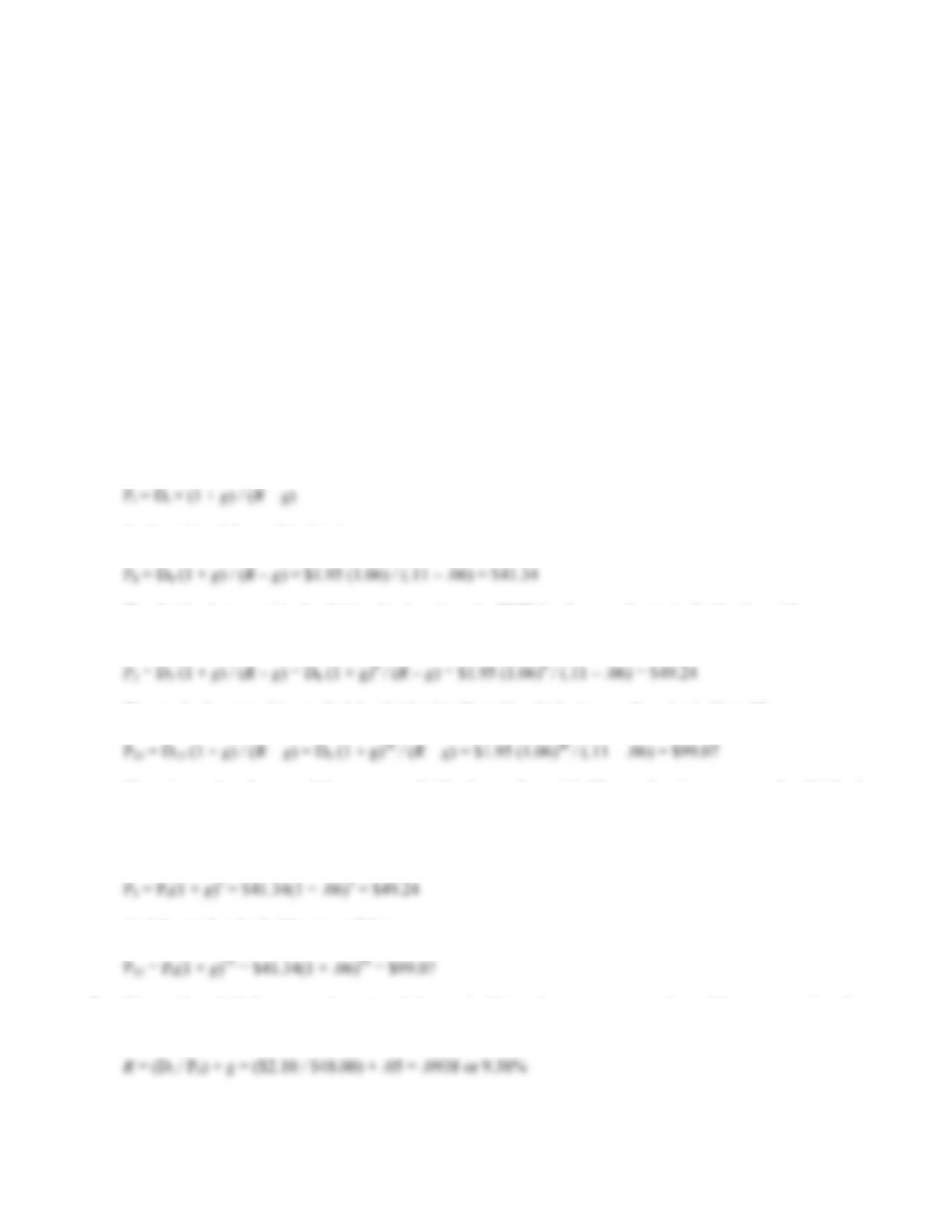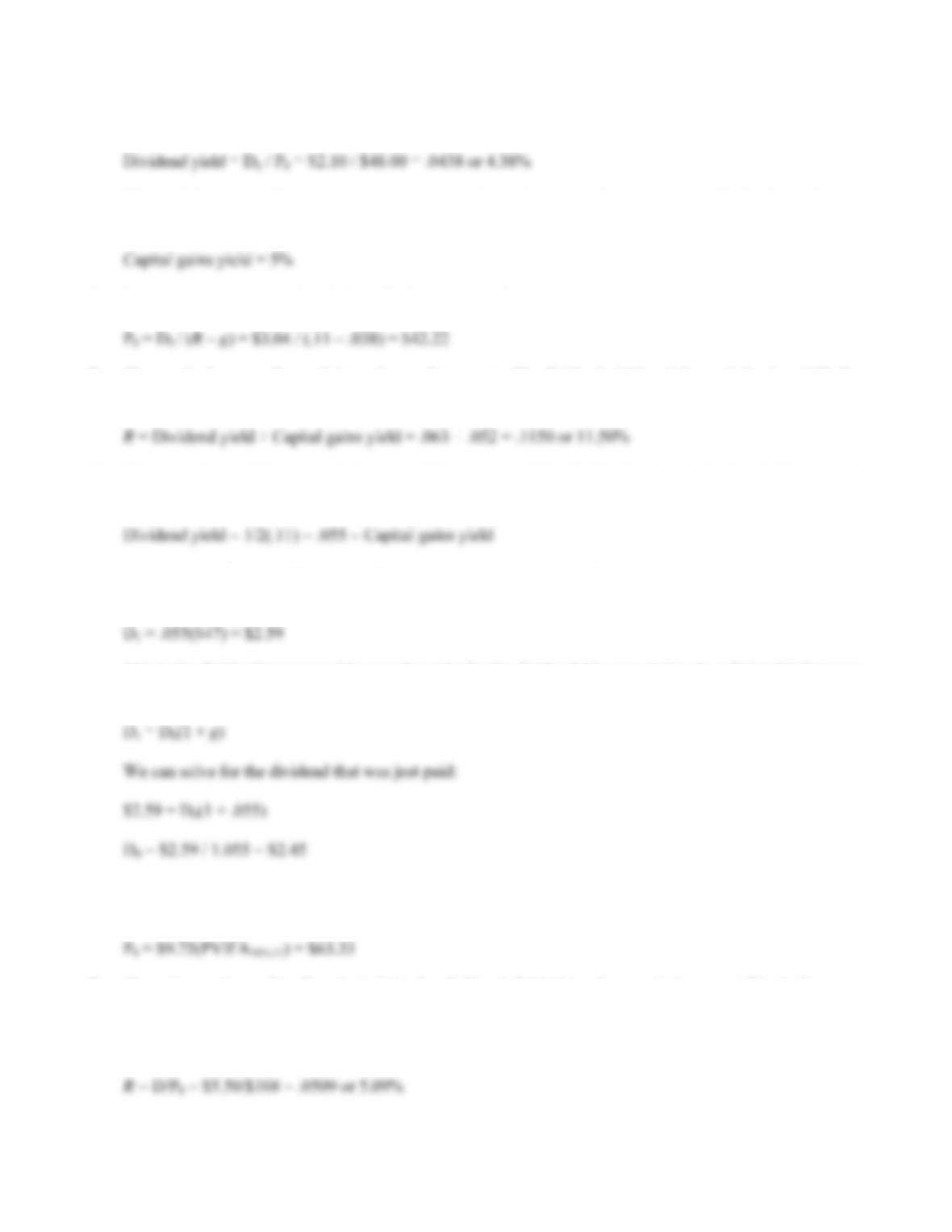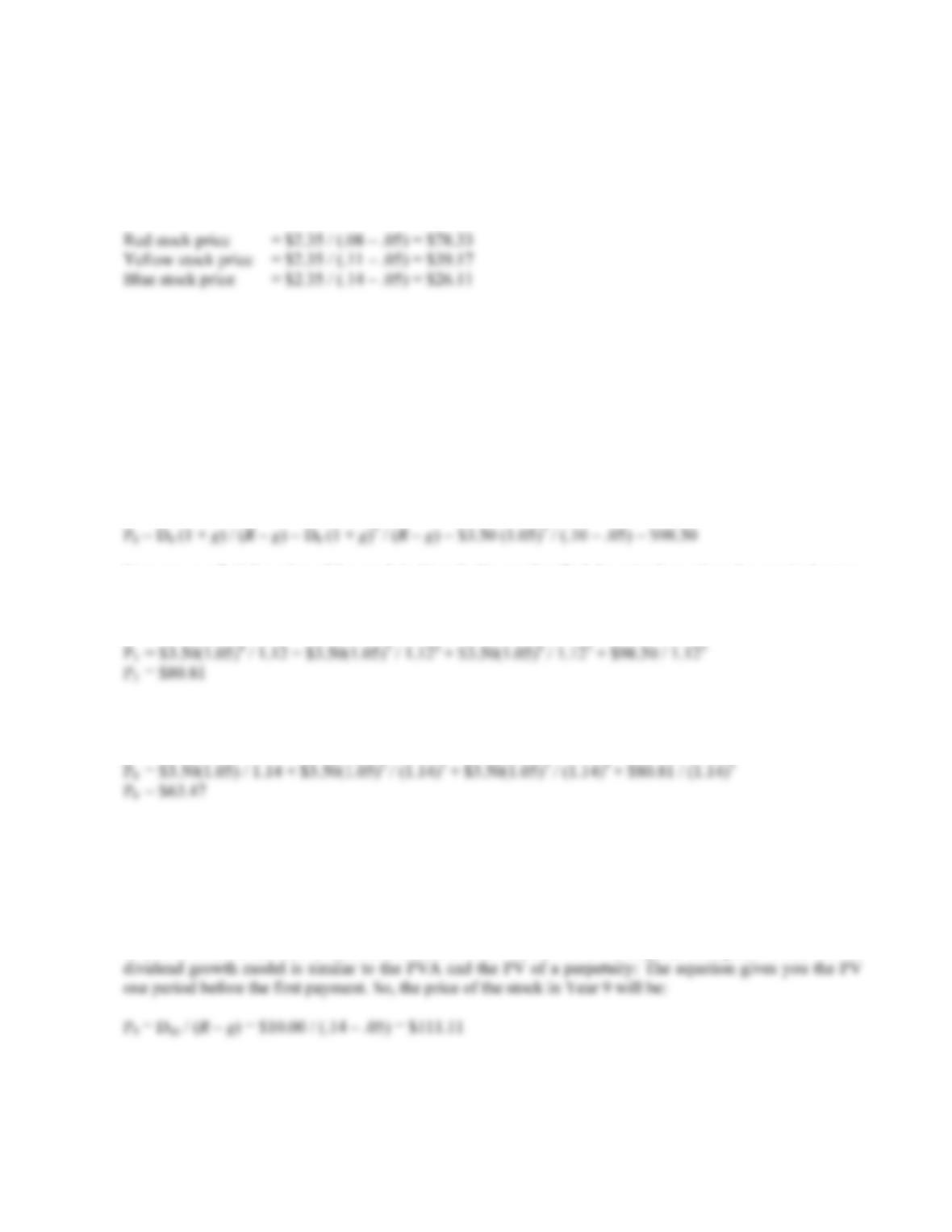Type
Quiz
Book Title
Fundamentals of Corporate Finance Standard Edition 9th Edition
ISBN 13
978-0073382395

### 978-0073382395 Chapter 8 Questions and Problems 1-10

April 3, 2019B-142 SOLUTIONS
11. Presumably, the current stock value reflects the risk, timing and magnitude of all future cash flows, both
short-term and long-term. If this is correct, then the statement is false.
12. If this assumption is violated, the two-stage dividend growth model is not valid. In other words, the price
calculated will not be correct. Depending on the stock, it may be more reasonable to assume that the
dividends fall from the high growth rate to the low perpetual growth rate over a period of years, rather than
in one year.
Solutions to Questions and Problems
NOTE: All end of chapter problems were solved using a spreadsheet. Many problems require multiple steps.
Due to space and readability constraints, when these intermediate steps are included in this solutions manual,
rounding may appear to have occurred. However, the final answer for each problem is found without rounding
during any step in the problem.
Basic
1. The constant dividend growth model is:
P
So the price of the stock today is:
P
The dividend at year 4 is the dividend today times the FVIF for the growth rate in dividends and four years,
so:
P
We can do the same thing to find the dividend in Year 16, which gives us the price in Year 15, so:
P
There is another feature of the constant dividend growth model: The stock price grows at the dividend
growth rate. So, if we know the stock price today, we can find the future value for any time in the future we
want to calculate the stock price. In this problem, we want to know the stock price in three years, and we
have already calculated the stock price today. The stock price in three years will be:
P
And the stock price in 15 years will be:
P
2. We need to find the required return of the stock. Using the constant growth model, we can solve the
equation for R. Doing so, we find:CHAPTER 8 B-143
3. The dividend yield is the dividend next year divided by the current price, so the dividend yield is:
The capital gains yield, or percentage increase in the stock price, is the same as the dividend growth rate,
so:
4. Using the constant growth model, we find the price of the stock today is:
P
5. The required return of a stock is made up of two parts: The dividend yield and the capital gains yield. So,
the required return of this stock is:
6. We know the stock has a required return of 11 percent, and the dividend and capital gains yield are equal,
so:
Now we know both the dividend yield and capital gains yield. The dividend is simply the stock price times
the dividend yield, so:
D
This is the dividend next year. The question asks for the dividend this year. Using the relationship between
the dividend this year and the dividend next year:
D
7. The price of any financial instrument is the PV of the future cash flows. The future dividends of this stock
are an annuity for 11 years, so the price of the stock is the PVA, which will be:
P
8. The price a share of preferred stock is the dividend divided by the required return. This is the same
equation as the constant growth model, with a dividend growth rate of zero percent. Remember, most
preferred stock pays a fixed dividend, so the growth rate is zero. Using this equation, we find the price per
share of the preferred stock is:B-144 SOLUTIONS
9. We can use the constant dividend growth model, which is:
P
t = Dt × (1 + g) / (Rg)
So the price of each company’s stock today is:
As the required return increases, the stock price decreases. This is a function of the time value of money: A
higher discount rate decreases the present value of cash flows. It is also important to note that relatively
small changes in the required return can have a dramatic impact on the stock price.
Intermediate
10. This stock has a constant growth rate of dividends, but the required return changes twice. To find the value
of the stock today, we will begin by finding the price of the stock at Year 6, when both the dividend growth
rate and the required return are stable forever. The price of the stock in Year 6 will be the dividend in Year
7, divided by the required return minus the growth rate in dividends. So:
P
Now we can find the price of the stock in Year 3. We need to find the price here since the required return
changes at that time. The price of the stock in Year 3 is the PV of the dividends in Years 4, 5, and 6, plus
the PV of the stock price in Year 6. The price of the stock in Year 3 is:
P
Finally, we can find the price of the stock today. The price today will be the PV of the dividends in Years
1, 2, and 3, plus the PV of the stock in Year 3. The price of the stock today is:
P
11. Here we have a stock that pays no dividends for 10 years. Once the stock begins paying dividends, it will
have a constant growth rate of dividends. We can use the constant growth model at that point. It is
important to remember that general constant dividend growth formula is:
P
t = [Dt × (1 + g)] / (Rg)
This means that since we will use the dividend in Year 10, we will be finding the stock price in Year 9. The